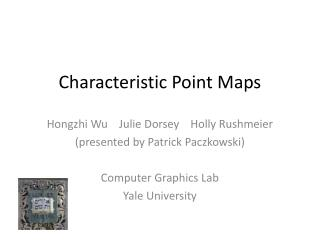DownloadDownload PresentationCharacteristic Point Maps

# Characteristic Point Maps

Télécharger la présentation## Characteristic Point Maps

- - - - - - - - - - - - - - - - - - - - - - - - - - - E N D - - - - - - - - - - - - - - - - - - - - - - - - - - -
##### Presentation Transcript

1. Characteristic Point Maps Hongzhi Wu Julie Dorsey Holly Rushmeier (presented by Patrick Paczkowski) Computer Graphics Lab Yale University

2. Outline • Introduction • Previous Work • Characteristic Point Maps • Derivation • Preprocessing • Usage • Results • Conclusions • Future Work

3. Motivation • Challenging to Render • Highly complex geometry + materials • High sampling rate to avoid aliasing • Viewed at multiple scales

4. Introduction • We present Characteristic Point Maps (CPMs) • A hierarchy of points on the original model • Preserves appearance (i.e. 6D filtered SV-BRDF) at multiple scales • Precomputed object-space adaptive sampling

5. Introduction level 0 level 1 level n Mesh Hierarchy Characteristic Point Maps …… original model simplified meshes …… Render Preprocess + Output Image Original Model

6. Previous Work • Texture Mipmap [Wil83] • Pre-filtering textures • Mesh Simplification [GH98, LT00, SSGH01] • Minimizing texture-mapping distortion • Appearance-Preserving Mesh Simplification [COM98] • Missing small-scale shadowing and masking effects • For textures, not general BRDFs • BTF LOD representation [MCT*05] • Dense sampling of 6D BTF for high-frequency effects

7. Previous Work

8. Derivations • Reflected radiance at x incident radiance BRDF reflected radiance visibility term cosine term ωo dL(x, ωo) ωi Li(x, ωi) x

9. Average reflected radiance ωo ωi avis A Avis

10. Derivations • After a sequence of transformations, where apparent reflectance function

11. Derivations • Average reflectance function • 6D function • Brute-force precomputation is impossible! • No analytical model => huge storage • E.g. 642 for A, 6x642 for both ωi and ωo • 642x(6x642)x(6x642) = 2473 Billion! • Difficult to compress numerically

12. Derivations Discretize integration into summation

13. Derivations Visible projected area term • Visible Projected Area Term • a 2D spherical function • Precompute on GPU and compress using Haar wavelets

14. Derivations Summation term • Summation Term • Want to reduce the number of items • (i.e. find the characteristic points) • Use Randomized Matrix Column Sampling

15. Illustration ωi1,ωo1 ωi2,ωo2 …… ωid,ωod x1 x2 …...

16. Illustration x1 x2 …... ωi1,ωo1 ωi2,ωo2 … …… ωid,ωod x1 x2 …...

17. Illustration x1 x2 …... ωi1,ωo1 … … … ωi2,ωo2 … …… ωid,ωod x1 x2 …...

18. Illustration …… x1 x2 …... ωi1,ωo1 α1 × + ωi2,ωo2 α2 × … + ωid,ωod α3 × x1 x2 …... α1 α2 α3

19. Randomized Matrix Column Sampling • Use [DMM06] to sample columns (i.e. to find characteristic points) • Compute a prob. distribution for choosing a column from the matrix • Randomly select m columns according to the prob. distribution • Compute the weights for these m columns

20. Randomized Matrix Column Sampling • Measure error as L2 norm • Iterate to “boost” the probability of getting the optimal result • Exploit spatial coherence in apparent reflectance functions • Determine the number of CPs as the minimum number to achieve certain approximation quality • High spatial coherence => small number of CPs • Low spatial coherence => large number of CPs

21. Preprocessing original model simplified meshes Mesh Hierarchy • Build a mesh hierarchy • Simplify geometry using existing techniques [GH97] • Establish a mapping from each simplified mesh to CPM u-v space Preprocess …… Original Model

22. Preprocessing original model simplified meshes level 0 level 1 level n Mesh Hierarchy Characteristic Point Maps • Build a CPM hierarchy • For each texel in CPM, we store • References to characteristic points • Corresponding weights • Wavelet coefficients for avis • Bottom-up construction Preprocess …… + Original Model …… α1 × + α2 × + α3 ×

23. Using CPMs • Select a simplified mesh • Select CPM mip level • Look up a particular texel • Evaluate at characteristic points ONLY!

24. Results: Cylinder Multi-sampled Normal Map Ground Truth CPMs Equal-time Budget ωi ωo

25. Results: Bolts Multi-sampled Normal Map Ground Truth CPMs Equal-time Budget

26. Results: Wall Multi-sampled Normal Map Ground Truth CPMs Equal-time Budget

27. Results: Gargoyles Close-up view Ground Truth CPMs Equal-time Budget

28. 46 Results • Precomputed object-space adaptive sampling • CP density adapts to the complexity of filtered SV-BRDFs 0

29. Conclusions • A general framework for efficiently computing and representing 6D spatially-varying average reflectance functions • No assumptions on geometry or BRDFs • Accelerates rendering • A precomputed object-space adaptive sampling method

30. Future Work • Apply a low-pass filter • Incorporate indirect illumination • Apply to deformable objects

31. Acknowledgements • National Science Foundation Grant #0528204 • Yale Graphics Group • Sumanta Pattanaik (UCF) • Li-Yi Wei (Microsoft) • Ping Tan (NUS)

32. Thank you • Questions? • Contact: hongzhi.wu@yale.edu

33. Back-up slides

34. Characteristic Point Maps Multi-sampled Normal Map Ground Truth Back-up slides (a) (b) (c) ωi ωo (d) (e) (f)